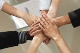# all rad grid properties are lost on postback

GridTop achievementsRank 1
Tharindu asked on 29 Oct 2013, 12:20 PM
Hi,

I have a rad grid where all the columns created dynamically from code behind. Some are template columns and others are bound columns. I have a save button which is outside the grid where i need to read the cell values on click event.
But inside the on click event I can't access the mastertableview columns or items.

Eg: radGrid.MasterTableView.Items.Count - this equals to zero. Therefore I can't iterate through the cell values.

The grid is defined on the markup. And I add the dynamically columns on Page_Init(). I'm assigning a dynamically generated DataTable as the datasource for the grid.

I have tried lot of solutions suggested in forums but nothing works. So now I started reading all the cell values from client side and it seems slow. Can somebody help?

Thanks.

## 1 Answer, 1 is accepted

0Princy
Top achievementsRank 1
answered on 30 Oct 2013, 07:19 AM
Hi Tharindu,

Please try the sample code snippet,I have added the grid and its columns from code behind,and on the button click event,I'm accessing all the cell value.Make sure you have the entire Radgrid created from code behind and you use the NeedDataSource event of the radgrid to populate the grid.

ASPX:
`<``asp:PlaceHolder` `ID``=``"PlaceHolder1"` `runat``=``"server"``></``asp:PlaceHolder``>`
`<``asp:Button` `ID``=``"Button1"` `runat``=``"server"` `Text``=``"Save"` `OnClick``=``"Button1_Click"` `/>`

C#:
`RadGrid grid;`
`protected` `void` `Page_Init(``object` `sender, System.EventArgs e)`
`{`
`    ``DefineGridStructure();`
`}`
`private` `void` `DefineGridStructure()`
`{`
`    ``grid = ``new` `RadGrid();`
`    ``grid.ID = ``"RadGrid1"``;  `
`    ``grid.Skin = ``"Vista"``;`
`    ``grid.Width = Unit.Percentage(100);`
`    ``grid.PageSize = 15;`
`    ``grid.AllowPaging = ``true``;`
`    ``grid.PagerStyle.Mode = GridPagerMode.NextPrevAndNumeric;`
`    ``grid.AutoGenerateColumns = ``false``;`
`    ``grid.AllowFilteringByColumn = ``true``;       `
`    ``grid.MasterTableView.Width = Unit.Percentage(100);`
`    ``grid.MasterTableView.DataKeyNames = ``new` `string``[] { ``"OrderID"` `};`
`    ``grid.NeedDataSource += ``new` `GridNeedDataSourceEventHandler(grid_NeedDataSource);   ``//Declaring the NeedDataSource  event`

`    ``//Creating BoundColumns`
`    ``GridBoundColumn boundColumn = ``new` `GridBoundColumn();`
`    ``grid.MasterTableView.Columns.Add(boundColumn);`
`    ``boundColumn.DataField = ``"OrderID"``;`
`    ``boundColumn.HeaderText = ``"OrderID"``;`
`    ``boundColumn.UniqueName = ``"OrderID"``;             `

`    ``boundColumn = ``new` `GridBoundColumn();`
`    ``grid.MasterTableView.Columns.Add(boundColumn);`
`    ``boundColumn.DataField = ``"ShipCity"``;`
`    ``boundColumn.HeaderText = ``"ShipCity"``;`
`    ``boundColumn.UniqueName = ``"ShipCity"``;`

`    ``GridDateTimeColumn date = ``new` `GridDateTimeColumn();`
`    ``grid.MasterTableView.Columns.Add(date);`
`    ``date.DataField = ``"OrderDate"``;`
`    ``date.HeaderText = ``"OrderDate"``;`
`    ``date.UniqueName = ``"OrderDate"``;`
`    ``date.DataFormatString = ``"{0:MM/dd/yy}"``;`
`    ``date.DataType = ``typeof``(System.DateTime);`
`    ``date.HeaderStyle.Width = Unit.Pixel(200);`
`    ``date.FilterControlWidth = Unit.Pixel(200);`

`    ``//Creating TemplateColumn`
`    ``string` `templateColumnName = ``"EmployeeId"``;`
`    ``GridTemplateColumn templateColumn = ``new` `GridTemplateColumn();`
`    ``templateColumn.ItemTemplate = ``new` `MyTemplate(templateColumnName);`
`    ``grid.MasterTableView.Columns.Add(templateColumn);`
`    ``templateColumn.HeaderText = templateColumnName;`
`    ``templateColumn.DataField = ``"EmployeeID"``;`
`       `
`    ``// Add the grid to the placeholder  `
`    ``this``.PlaceHolder1.Controls.Add(grid);`
`}`

`void` `grid_NeedDataSource(``object` `sender, GridNeedDataSourceEventArgs e)`
`{`
`    ``grid.DataSource = GetDataTable(``"SELECT * FROM Orders"``);`
`}`
`public` `class` `MyTemplate : ITemplate`
`{`
`    ``private` `string` `colname;`
`    ``protected` `Label lControl;`
`    ``public` `MyTemplate(``string` `cName)`
`    ``{`
`        ``colname = cName;`
`    ``}`
`    ``public` `void` `InstantiateIn(System.Web.UI.Control container)`
`    ``{`
`        ``lControl = ``new` `Label();`
`        ``lControl.ID = ``"lControl"``;`
`        ``lControl.DataBinding += ``new` `EventHandler(lControl_DataBinding);`
`        ``container.Controls.Add(lControl);`
`    ``}`

`    ``public` `void` `lControl_DataBinding(``object` `sender, EventArgs e)`
`    ``{`
`        ``Label l = (Label)sender;`
`        ``GridDataItem container = (GridDataItem)l.NamingContainer;`
`        ``l.Text = ((DataRowView)container.DataItem)[colname].ToString();`
`    ``}`
`}`
`public` `DataTable GetDataTable(``string` `query)`
`{`
`    ``String ConnString = ConfigurationManager.ConnectionStrings[``"Northwind_newConnectionString3"``].ConnectionString;`
`    ``SqlConnection conn = ``new` `SqlConnection(ConnString);`
`    ``SqlDataAdapter adapter = ``new` `SqlDataAdapter();`
`    ``adapter.SelectCommand = ``new` `SqlCommand(query, conn);`

`    ``DataTable myDataTable = ``new` `DataTable();`

`    ``conn.Open();`
`    ``try`
`    ``{`
`        ``adapter.Fill(myDataTable);`
`    ``}`
`    ``finally`
`    ``{`
`        ``conn.Close();`
`    ``}`

`    ``return` `myDataTable;`
`}`

`protected` `void` `Button1_Click(``object` `sender, EventArgs e)`
`{`
`    ``foreach` `(GridDataItem item ``in` `grid.MasterTableView.Items)`
`    ``{`
`        ``//Accessing BoundColumn values`
`        ``string` `id = item[``"OrderID"``].Text;`
`        ``string` `city = item[``"ShipCity"``].Text;`
`        ``string` `date = item[``"OrderDate"``].Text;`
`        ``//Accessing Template label control`
`        ``Label lbl = (Label)item.FindControl(``"lControl"``); `
`        ``string` `employeeId = lbl.Text;`
`    ``}`
`}`

Thanks,
Princy
Tags
GridRank 1Rank 1